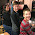## Monday, February 15, 2016

### Divisibility by Prime Factors and Seven

This post will just be some of my thoughts on teaching a couple of the divisibility presentations.  I am no mathematician so you may not want to do things the way I did and this may have all been obvious to you from the start.  These are just some changes I made to the instructions in our albums based on my understanding of things.

After teaching (and in most cases proving, using golden beads) the divisibility rules for 2, 5, 25, 4, 8, 3, 9, 11, and 7 I wanted to revisit the lesson on "divisibility by prime factors" as discussed here because I wasn't happy with the focus of that lesson.  In that presentation we recognize that a number is divisible by each its prime factors and any product thereof.  My kids thought that was kind of silly because in order to create a factor tree they had to determine which prime numbers the composite numbers could be divided by.  This is why I wondered if some of the advanced work on factors should be completed after divisibility.  In that work, my children were unable to complete the factor trees without my assistance (or a calculator) because they didn't know the rules for divisibility.  You would need either abstract long division skills (they are NOT pulling out racks and tubes to make a factor tree) or know the the divisibility rules to find the prime factors in that post.  Anyway, I felt the "divisibility by prime factors" presentation focuses on revealing the reciprocal relationship between factors and divisibility. While some students may need that presented, I think that stops short.  The most useful part of that tidbit of informations is that you can determine divisibility in the absence of a rule or shortcut using the prime factors.

We revisited this idea by asking if 32,352 was divisible by 24. Do we have a rule for 24?  No.  But we do know that a number is divisible by each its prime factors and any product thereof.  We can find the prime factors of 24 (2 and 3) and if 32,352 is divisible by both of those numbers it is also divisible by 24.  We do know divisibility rules for both 2 and 3 so the boys were able to determine divisibility quickly by applying those rules.

We practiced this again by asking if 151, 375 was divisible by 35.  We do not have a rule for 35 but we found it's prime factors (5 and 7) and applied the rules of divisibility for both of those numbers.

The math albums I have only taught one rule for divisibility by seven but I prefer to use two rules. The rule I found in the albums was the rule that "if the difference of the sum of the odd number periods and sum of even number periods is divisible by seven then the number is divisible by seven."
That sounded like Greek to me the first time I read it not realizing that what was meant by "periods" was a family of numbers between the commas in a large number (unit family, thousand family, million family, billion family).  So if you have a number like 654,076,790,241 you would add the unit and million family (076 + 241) and add the thousand family and billion family (654 +241) and find the difference between the two sums.  The problem is the difference is 1127 and my youngest child didn't know if that was divisible by seven either (you could if they are proficient at abstract long division).  This is where the other rule comes in handy.    You can double the last digit (7*2=14) and then subtract that product from the remaining digits of the number (112-14=98).  You can keep reapplying this rule as necessary until you find a number small enough to determine whether seven is a factor (see the link).  98 is divisible by seven.  So I find that neither of these rules are enough on their own.  Finding the difference between the odd and even number periods left us with a number still too large to determine divisibility without using abstract long division.  You would have to apply the second rule ten times to determine divisibility which is just too many. So our family uses the first rule for large numbers, the second rule for smaller numbers and combine them if necessary.

So for our question that day, is 151,375 divisible by 35, we used both rules.

375-151=224
4*2=8
22-8=14

14 is divisible by 7

1.1.2.